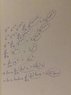# Figuring symmetries of a differential operator from its eigenfunctions

JPaquim
So, I understand that the derivative operator, $D=\frac{d}{dx}$ has translational invariance, that is: $x \rightarrow x - x_0$, and its eigenfunctions are $e^{\lambda t}$. Analogously, the theta operator $\theta=x\frac{d}{dx}$ is invariant under scalings, that is $x \rightarrow \alpha x$, and its eigenfunctions are $x^\lambda$. Taking logarithms and exponentials, I have constructed a sequence of operators and their respective eigenfunctions, all with the property that $\{L(\frac{d}{dx})\}f^\lambda(x)=\lambda f^\lambda(x)$. I've taken a picture and attached it to this post.

My guess is that associated with every single one of these operators is some symmetry, some sort of coordinate transformation $x \rightarrow f(x)$ under which the operator is invariant. For the $x\log x \frac{d}{dx}$ operator, its invariant under $x \rightarrow x^k$, by inspection. How can I figure out what sort of symmetry a given operator has, given its eigenfunctions?

Physically, symmetries are associated with conservation laws. For a system whose differential equations are governed by this sort of differential operators, what sort of conserved quantities should I expect?

#### Attachments

•IMG_0596.jpg
16.5 KB · Views: 375